﻿ A Modified Nadaraya-Watson Estimator for the Variance of the Finite Population MeanPublications are Open
Access in this journal
Article Versions
Export Article
• Normal Style
• MLA Style
• APA Style
• Chicago Style
Research Article
Open Access Peer-reviewed

### A Modified Nadaraya-Watson Estimator for the Variance of the Finite Population Mean

Charlotte K Mokaya , DR. Edward Gachangi Njenga
American Journal of Applied Mathematics and Statistics. 2019, 7(4), 146-151. DOI: 10.12691/ajams-7-4-4
Received May 06, 2019; Revised June 15, 2019; Accepted July 01, 2019

### Abstract

The main objective of this study was to derive a nonparametric estimator for the variance of the population mean when the population structure is nonlinear and heteroscedastic. Therefore, this paper sought to investigate the performance of Nadaraya-Watson estimator with a variable bandwidth. The methodology was derived by modifying the Nadaraya-Watson estimator where the bandwidth was a function of the range of observations. The performance of the proposed estimator was compared with other estimators i.e. Ratio estimator and Nadaraya-Watson with a fixed bandwidth. To measure performance of each of the estimators, average mean squared error was considered. It was found out that the Ratio estimator performs well for linear and homoscedastic populations while the Nadaraya-Watson with fixed bandwidth performs well for nonlinear and heteroscedastic populations. However, in the light of these findings, Nadaraya-Watson estimator (with variable bandwidth) was found to perform better and most efficient than the Ratio estimator and Nadaraya-Watson estimator (with fixed bandwidth) in nonlinear and heteroscedastic populations. It was also found to be the most robust compared to the estimators considered in this study.

### 1. Introduction

The concept of sample survey involves obtaining information regarding the population under study and subsequently making inferences about it.

Variance estimation of a population mean in sample survey is important as it gives further information about the accuracy of the estimators. According to the works of 1, the variance estimator of the population mean is also useful in the construction of confidence intervals and hypothesis testing.

However, variance estimation parameter can be unreliable when probability sampling is used for small sample sizes. Therefore, the use of auxiliary variables is considered as it gives more information about the population. According to 2, the incorporation of auxiliary information increases precision of the estimators. Presence of auxiliary variables provides good results compared to design-based techniques. On the other hand, use of auxiliary variables requires a model and assumptions to be specified.

In this paper, linearity and homoscedasticity assumptions were considered. Estimation of the parameter using an auxiliary variable yielded accurate results when the underlying assumptions are satisfied. On the contrary, when these assumptions are violated, estimators like the Ratio estimator becomes inefficient. This leads to inaccurate computation of the confidence intervals and wrong interpretation of the results is likely to occur. Furthermore, inaccurate results of the variance lead to wrong hypothesis testing and incorrect inference of population parameters.

Therefore, statisticians resorted to the use of non-parametric estimators which are robust when linearity and homoscedasticity assumptions are violated. Examples of some nonparametric estimators include; spline functions, local polynomial regression estimator and Nadaraya-Watson estimator with fixed bandwidth. Nonparametric variance estimation has been studied by 3 and 4, among others.

In this paper, a nonparametric estimator of the population variance is proposed which uses Nadaraya-Watson estimator with variable bandwidth.

### 2. Review of Nadaraya-Watson Estimator with Fixed Bandwidth

Nadaraya-Watson estimator is a nonparametric estimator proposed independently by 5 and 6 to estimate the mean function of a model using a sample of sizeWatson independently proposed a simple computer method for obtaining a graph from a large number of observations while Nadaraya proposed an estimator for approximating the regression curve as per 5 and 6. This estimator is based on locally weighted averaging.

Consider a random sampleof size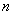with variables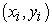…,and a joint probability distribution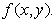Let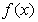be the probability density function ofConsider a nonparametric model of the form;(1)

where m(x) is an unknown regression function and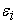are independent random errors with a mean of zero and variance ofAccording to 5 and 6, the estimator of the mean functionis given as;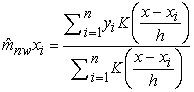(2)

where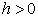is a fixed bandwidth parameter that controls the degree of smoothness of in equation (2) andis the kernel function. According to 7, a kernel is a piecewise continuous function that is symmetrical at zero and integrates to 1;Population mean and variance using the Nadaraya-Watson mean function with fixed bandwidth in equation (2) above was proposed by 8 and is as shown below;(3)

And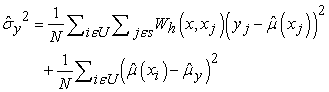(4)

where,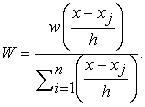In the next section, we derive Nadaraya-Watson estimator with a variable bandwidth. Let us call it a modified Nadaraya-Watson estimator.

### 3. Modified Nadaraya-Watson Estimator of the mean and variance functions.

In this paper, we derived an estimator of the variance of the population mean using the modified Nadaraya-Watson mean function proposed by 9. 9, modified Nadaraya-Watson mean function using a bandwidth which is a function of the range of observations. This was aimed at improving the performance of the estimator as well as making it more stable.

Letbe a pair of variables of a sample of sizewhere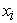is an auxiliary variable andis the study variable. These variables are positively related with a joint probability distribution function (pdf)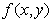can defined as=whereis the marginal density of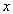andis the marginal density ofThe density functions as follows;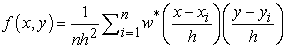(5)

and(6)

Using nonparametric equation (1), the residual termis estimated as below;(7)

Taking expectation on both sides of equation (7), we get,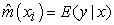(8)

From the expression in equation (8), the modified Nadaraya-Watson mean function is derived as below;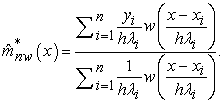(9)

whereis the bandwidth parameter andis defined as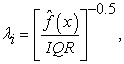whereby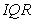is the interquartile range of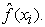The Interquartile range is used because it is not affected by the extreme values in the data.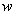denotes a smoothing parameter with the following properties;

i)ii)(10)

iii)Taking the square of the residual term in equation (7), we getTaking expectation of this expression, we get(11)

Taking expectation of this expression, we get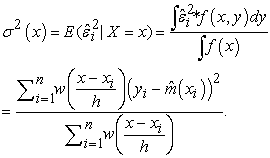(12)

Consider equation (12) and the proposed variance estimator for modified Nadaraya-Watson mean function in equation (9),is given as;(13)

where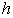denotes the bandwidth parameter andis the smoothing parameter that has the same properties asspecified in equation (10) and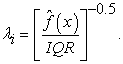3.2. Modified Nadaraya-Watson Estimates of the Finite Population Mean and Variance

In this section, we derived population mean and variance using the modified Nadaraya-Watson mean and variance functions obtained in section (3.1).

Population mean function is defined as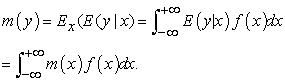(14)

The estimate of this population mean function given in (14) is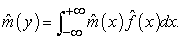(15)

The population variance function is defined as below;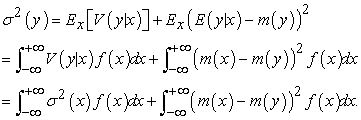(16)

and the estimate of the variance function in equation (16) is given as(17)

Combining equation (9) and (13) we get the proposed modified Nadaraya-Watson estimators of the population mean and variance as below;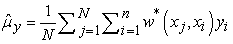(18)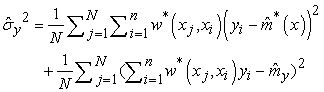(19)

where,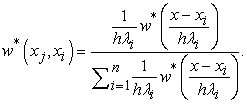### 4. Simulation Studies

Performance of the three estimators, that is, the Ratio estimator, Nadaraya-Watson with fixed bandwidth and the modified Nadaraya-Watson was compared using six simulated populations and one natural population. The average mean squared error criterion was used to measure efficiency of the estimators.

4.1. Description of the Study Population and Estimators

Below is the description of the populations where linear and quadratic equations were considered. The equations from the study took the below forms;

Linear Equation:Quadratic Equation: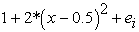where the auxiliary variable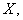was simulated from the uniform distribution with the interval [0,1];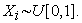.

The error term was simulated from the normal distribution with mean (0) and variance of (0.1);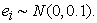4.2. Description of the Computation Procedure

A population of size N=10,000 was simulated using R software. 1000 samples of size n=500 were selected using simple random sampling without replacement.

The Gaussian Kernel function defined as;where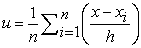was used in the study for fixed and modified Nadaraya-Watson estimators.

The performance of the kernel function depends on the choice of the bandwidth parameter. Choosing a bandwidth that balances the variance with the bias is crucial. According to 10, choice of the bandwidth can be done by data analysts either subjectively or objectively. In this research, fixed bandwidthdefined below, was obtained from unbiased (Least square) cross-validation method whose equation is as below;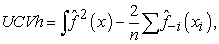whereis the number of observations andis the density estimate without data pointThe smoothing parameteris obtained by minimizingAverage bias was obtained using the below equation;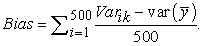where k denotes different estimators.

Average MSE was obtained using equation;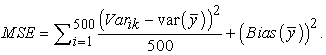Relative change of efficiency (RCE) was also calculated from the equation below;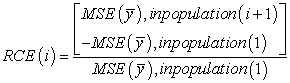=Ratio estimator, fixed Nadaraya-Watson and modified Nadaraya-Watson estimators.

The following are scatter plots showing distributions of seven populations analyzed.

• Figure 1. Linear population with homoscedastic variance structure
• Figure 2. Quadratic population with homoscedastic variance structure
• Figure 3. Linear population with heteroscedastic variance structure
• Figure 4. Population is quadratic with heteroscedastic variance structure
• Figure 5. Linear population with heteroscedastic variance structure
• Figure 6. Population is nonlinear and heteroscedastic
• Figure 7. Real population that is neither linear nor homoscedastic
4.3. Results and Interpretations

In Table 2, Ratio estimator has the smallest variance for populations I, III and V followed by Nadaraya-Watson with fixed bandwidth estimator. Modified Nadaraya-Watson estimator comes in last. For populations II, IV, VI and VII which are nonlinear homoscedastic and nonlinear heteroscedastic, our proposed estimator has the smallest variance estimate followed by Nadaraya-Watson estimator with fixed bandwidth. Ratio estimator comes in third.

The squared average mean errors of the estimators are calculated to assess their efficiency. The results are as shown in Table 3. In light of the statistics and tests above, modified Nadaraya-Watson is most efficient in populations with nonlinear structure compared to the other two estimators. Therefore, modified Nadaraya-Watson estimator is most robust when the linear structure of the populations is violated.

### 5. Conclusion

Following the results of our data analysis, we noted that the Ratio estimator performs well for linear and homoscedastic model but when there is violation of the model structure, the estimator breaks down. Therefore, it can be concluded that Ratio estimator is not efficient when linear and homoscedastic assumptions of a population are violated. Nadaraya-Watson with fixed bandwidth and Nadaraya-Watson with variable bandwidth estimators performed well in nonlinear heteroscedastic populations. However, the proposed estimator performed even better compared to the Nadaraya-Watson with fixed bandwidth as it was the most efficient amongst the estimators considered in this study.

### References

  Yuejin, Z., Yebin, C. and Tiejun, T. (2014). A Least Squares Method for Variance Estimation in Heteroscedastic Nonparametric Regression. Hindawi Publishing Corporation, 1-14. In article View Article  Wu, C. and Sitter, R. (2001). Variance estimation for the finite population distribution function with complete auxiliary information.The Canadian Journal of Statistics, 29, 289-307. In article View Article  Hall, P. and Marron, J.S. (1990). On Variance estimation in Nonparametric Regression. Biomerika, 77, 415-419. In article View Article  Shen, S. and Mei, C. (2009). Estimation of the Variance Function in Heteroscedastic Linear Regression Models. Communications in Statistics – Theory and Methods, 38, 1098-1112. In article View Article  Nadaraya, E.A. (1964). On Estimating Regression. Theory of probability application, 9,141-142. In article View Article  Watson, G. (1964). Smooth Regression Analysis. Sankhya, 26, 359-372. In article  Hardle, W. (1994). Applied Nonparametric Regression. Cambridge University Press. In article View Article  Njenga, E. and Smith, T.M.F. (1992). Robust Model-Based Methods for Analytic Surveys. Survey Methodology, 18,187-208. In article  Aljuhan, H. and Alturuk, I. (2014). Modification of the Adaptive Nadaraya-Watson Kernel Regression Estimator. Academic Journals, 9, 966-971. In article View Article  Fan and Irene, G. (1992). Variable Bandwidth and Local Linear Regression Smoothers. The Annals of Statistics, 20, 2008-2036. In article View Article

Published with license by Science and Education Publishing, Copyright © 2019 Charlotte K Mokaya and DR. Edward Gachangi NjengaThis work is licensed under a Creative Commons Attribution 4.0 International License. To view a copy of this license, visit http://creativecommons.org/licenses/by/4.0/